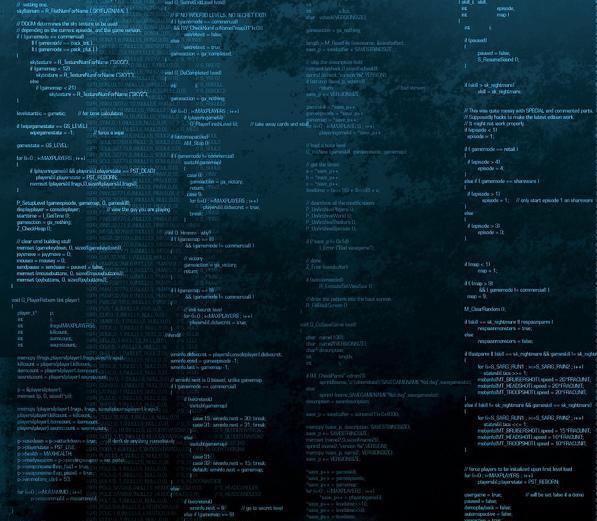# What Is Floyd's Triangle?

C. Mitchell

Floyd’s triangle is a series of numbers that are sequentially spread across a series of rows. It is used to teach computer programming basics. The first row contains a 1 by itself, and the second row contains 2 and 3. The next row holds 4, 5 and 6, and the numbers continue in this pattern infinitely. A right triangle results, with numerals spaced at even intervals.Floyd's triangle is often taught along with Pascal's triangle, invented by Blaise Pascal.

The form of Floyd’s triangle is not complicated. Most of the trick is in designing a program to generate the numbers in order and with the proper spacing, with only minimal commands. Computer programming instructors teaching both Java and C++ frequently assign Floyd’s triangle problems to students to teach fundamental programming principles.In C++ coding, loops are code structures that depend on statements or groups of statements being executed multiple times.

Building the triangle’s formula involves complex math and integer solving skills that are essential in larger programming projects. Each progressive row of the triangle builds on the prior, but is not a sum total. To generate a computer program that will systematically build the triangle out to a certain specified size, students must understand integer mathematics and apply it to the script language and unique lexicon of computer coding.

Properly coding Floyd’s triangle requires a mastery of loops. In C++ and Java coding, loops are code structures that depend on statements or groups of statements being executed multiple times. The statement must contain an undefined integer that becomes defined in a unique way with each loop.

Floyd’s triangle also contains mathematical significance outside of the programming sector. Aside from being an exponentially expanding perfect right triangle, it also defines both triangular numbers and the numbers that make up the “lazy caterer’s sequence.” Both are facets of polynomials and geometric calculations.

Triangular numbers are the numbers that result when sequential numbers are serially added together. The calculation begins with 1, which is the first triangular number. Then, 1+2=3, making 3 the second triangular number; that whole calculation is then added to the next number, generating (1+2)+3=6. From there, (1+2+3)+4=10, and so on. Not coincidentally, the numbers 1, 3, 6 and 10 are on the right-hand edge of Floyd’s triangle.

The left-hand edge contains the numbers of the lazy caterer’s sequence. That sequence describes the maximum number of pieces that can result when straight lines are used to bisect a circle. Pieces need not be equal, because lines do not have to pass directly through the center’s circle. Possible numbers can be generated with the formula (n2 + n + 2)/2, which yields a list that starts with 1, 2, 4, 7, and 11 — the numbers at the start of the first five rows of Floyd’s triangle.

Math instructors often teach Floyd’s triangle alongside Pascal’s triangle, which is another collection of ordered numbers that sheds light on various of mathematical patterns and formulas. Pascal’s triangle is an equilateral triangle made up of building binomial coefficients. This triangle also can be coded in computer programming, although the programming that is required usually is more advanced than the programming that is needed for Floyd’s model.

## You might also Like